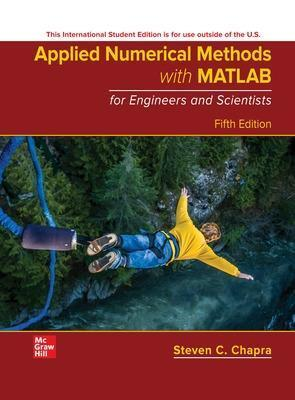# APPLIED NUMERICAL METHOD WTIH MATLAB FOR ENGINEERS AND SCIENTISTS 5th ISE - STEVEN CHAPRA 9781265148225

RM 140.00

TITLE :APPLIED NUMERICAL METHOD WTIH MATLAB FOR ENGINEERS AND SCIENTISTS - STEVEN CHAPRA

ISBN13 : 9781265148225

PUBLISHER : MCGRAWHILL (2022)

EDITION : 5E ISE PAPERBACK

PAGES :  732 PAGES

Applied Numerical Methods with MATLAB is designed to support a one-semester course in numerical methods. It has been written for students who want to learn and apply numerical methods in order to solve problems in engineering and science. As such, the methods are motivated by problems rather than by mathematics. That said, sufficient theory is provided so students come away with insight into the techniques and their shortcomings.

1 Mathematical Modeling, Numerical Methods, and Problem Solving
2 MATLAB Fundamentals
3 Programming with MATLAB
4 Roundoff and Truncation Errors
5 Roots: Bracketing Methods
6 Roots: Open Methods
7 Optimization
8 Linear Algebraic Equations and Matrices
9 Gauss Elimination
10 LU Factorization
11 Matrix Inverse and Condition
12 Iterative Methods
13 Eigenvalues
14 Linear Regression
15 General Linear Least-Squares and Nonlinear Regression
16 Fourier Analysis
17 Polynomial Interpolation
18 Splines and Piecewise Interpolation
19 Numerical Integration Formulas
20 Numerical Integration of Functions
21 Numerical Differentiation
22 Initial-Value Problems
23 Adaptive Methods and Stiff Systems
24 Boundary-Value Problems